# 量子相位估计

## 1.1 直觉

(在傅里叶基中)到计数寄存器中的 t 个量子位。然后我们使用逆QFT将其从傅里叶基转化为我们可以测量的计算基。

### 1.2数学基础

!. 设置: \vert\psi\rangle 在一组量子比特寄存器中。计数寄存器中另一组 n 量子比特，我们将在其上存储 2^n\theta :

|\psi_0\rangle = \lvert 0 \rangle^{\otimes n} \lvert \psi \rangle

!!. 叠加:在计数寄存器上应用 n 位的哈达玛门操作 H^{\otimes n} :

|\psi_1\rangle = {\frac {1}{2^{\frac {n}{2}}}}\left(|0\rangle +|1\rangle \right)^{\otimes n} \lvert \psi \rangle

!!!. 受控酉操作:我们需要引入受控酉操作 CU ，它只在目标寄存器对应的控制位为 |1\rangle 时对其应用酉操作符 U 。由于 U 是一个具有特征向量 |\psi\rangle 的酉算子，使得 U|\psi \rangle =e^{\boldsymbol{2\pi i} \theta}|\psi \rangle ，这意味着:

U^{2^{j}}|\psi \rangle =U^{2^{j}-1}U|\psi \rangle =U^{2^{j}-1}e^{2\pi i\theta }|\psi \rangle =\cdots =e^{2\pi i2^{j}\theta }|\psi \rangle

\begin{aligned} |\psi_{2}\rangle & =\frac {1}{2^{\frac {n}{2}}} \left(|0\rangle+{e^{\boldsymbol{2\pi i} \theta 2^{n-1}}}|1\rangle \right) \otimes \cdots \otimes \left(|0\rangle+{e^{\boldsymbol{2\pi i} \theta 2^{1}}}\vert1\rangle \right) \otimes \left(|0\rangle+{e^{\boldsymbol{2\pi i} \theta 2^{0}}}\vert1\rangle \right) \otimes |\psi\rangle\\\\ & = \frac{1}{2^{\frac {n}{2}}}\sum _{k=0}^{2^{n}-1}e^{\boldsymbol{2\pi i} \theta k}|k\rangle \otimes \vert\psi\rangle \end{aligned}

iv. 傅里叶逆变换:请注意，上面的表达式正是应用量子傅里叶变换的结果，就像我们在量子傅里叶变换及其Qiskit实现的笔记本上推导的那样。回想一下，QFT将n个量子比特的输入状态 \vert x\rangle 映射到输出为

QFT\vert x \rangle = \frac{1}{2^\frac{n}{2}} \left(\vert0\rangle + e^{\frac{2\pi i}{2}x} \vert1\rangle\right) \otimes \left(\vert0\rangle + e^{\frac{2\pi i}{2^2}x} \vert1\rangle\right) \otimes \ldots \otimes \left(\vert0\rangle + e^{\frac{2\pi i}{2^{n-1}}x} \vert1\rangle\right) \otimes \left(\vert0\rangle + e^{\frac{2\pi i}{2^n}x} \vert1\rangle\right)

\vert\psi_3\rangle = \frac {1}{2^{\frac {n}{2}}}\sum _{k=0}^{2^{n}-1}e^{\boldsymbol{2\pi i} \theta k}|k\rangle \otimes | \psi \rangle \xrightarrow{\mathcal{QFT}_n^{-1}} \frac {1}{2^n}\sum _{x=0}^{2^{n}-1}\sum _{k=0}^{2^{n}-1} e^{-\frac{2\pi i k}{2^n}(x - 2^n \theta)} |x\rangle \otimes |\psi\rangle

v. 测量:上面的表达式在 x = 2^n\theta 附近达到峰值。对于 2^n\theta 为整数的情况，在计算基中测量会以高概率给出辅助寄存器中的相位:

|\psi_4\rangle = | 2^n \theta \rangle \otimes | \psi \rangle

## 2. 示例:t型门

T|1\rangle = \begin{bmatrix} 1 & 0\\ 0 & e^\frac{i\pi}{4}\\ \end{bmatrix} \begin{bmatrix} 0\\ 1\\ \end{bmatrix} = e^\frac{i\pi}{4}|1\rangle

T|1\rangle = e^{2i\pi\theta}|1\rangle

\theta = \frac{1}{8}

## 2.1创建电路


#initialization

import matplotlib.pyplot as plt

import numpy as np

import math

# importing Qiskit

from qiskit import IBMQ, Aer, transpile, assemble

from qiskit import QuantumCircuit, ClassicalRegister, QuantumRegister

# import basic plot tools

from qiskit.visualization import plot_histogram




qpe = QuantumCircuit(4, 3)

qpe.x(3)

qpe.draw()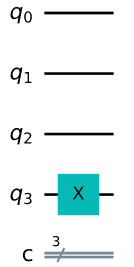for qubit in range(3):

qpe.h(qubit)

qpe.draw()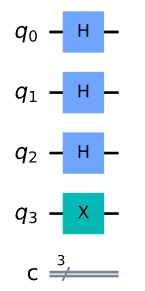repetitions = 1

for counting_qubit in range(3):

for i in range(repetitions):

qpe.cp(math.pi/4, counting_qubit, 3); # This is CU

repetitions *= 2

qpe.draw()




def qft_dagger(qc, n):

"""n-qubit QFTdagger the first n qubits in circ"""

# Don't forget the Swaps!

for qubit in range(n//2):

qc.swap(qubit, n-qubit-1)

for j in range(n):

for m in range(j):

qc.cp(-math.pi/float(2**(j-m)), m, j)

qc.h(j)




qpe.barrier()

# Apply inverse QFT

qft_dagger(qpe, 3)

# Measure

qpe.barrier()

for n in range(3):

qpe.measure(n,n)



qpe.draw()



## 2.2结果


aer_sim = Aer.get_backend('aer_simulator')

shots = 2048

t_qpe = transpile(qpe, aer_sim)

qobj = assemble(t_qpe, shots=shots)

results = aer_sim.run(qobj).result()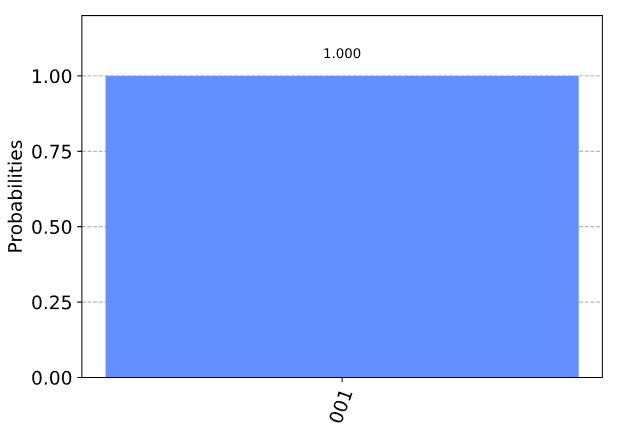\theta = \frac{1}{2^3} = \frac{1}{8}

## 3.示例:提高精度

### 3.1问题


# Create and set up circuit

qpe2 = QuantumCircuit(4, 3)

# Apply H-Gates to counting qubits:

for qubit in range(3):

qpe2.h(qubit)

# Prepare our eigenstate |psi>:

qpe2.x(3)

# Do the controlled-U operations:

angle = 2*math.pi/3

repetitions = 1

for counting_qubit in range(3):

for i in range(repetitions):

qpe2.cp(angle, counting_qubit, 3);

repetitions *= 2

# Do the inverse QFT:

qft_dagger(qpe2, 3)

# Measure of course!

for n in range(3):

qpe2.measure(n,n)

qpe2.draw()




# Let's see the results!

aer_sim = Aer.get_backend('aer_simulator')

shots = 4096

t_qpe2 = transpile(qpe2, aer_sim)

qobj = assemble(t_qpe2, shots=shots)

results = aer_sim.run(qobj).result()### 3.2解决方案


# Create and set up circuit

qpe3 = QuantumCircuit(6, 5)

# Apply H-Gates to counting qubits:

for qubit in range(5):

qpe3.h(qubit)

# Prepare our eigenstate |psi>:

qpe3.x(5)

# Do the controlled-U operations:

angle = 2*math.pi/3

repetitions = 1

for counting_qubit in range(5):

for i in range(repetitions):

qpe3.cp(angle, counting_qubit, 5);

repetitions *= 2

# Do the inverse QFT:

qft_dagger(qpe3, 5)

# Measure of course!

qpe3.barrier()

for n in range(5):

qpe3.measure(n,n)

qpe3.draw()




# Let's see the results!

aer_sim = Aer.get_backend('aer_simulator')

shots = 4096

t_qpe3 = transpile(qpe3, aer_sim)

qobj = assemble(t_qpe3, shots=shots)

results = aer_sim.run(qobj).result()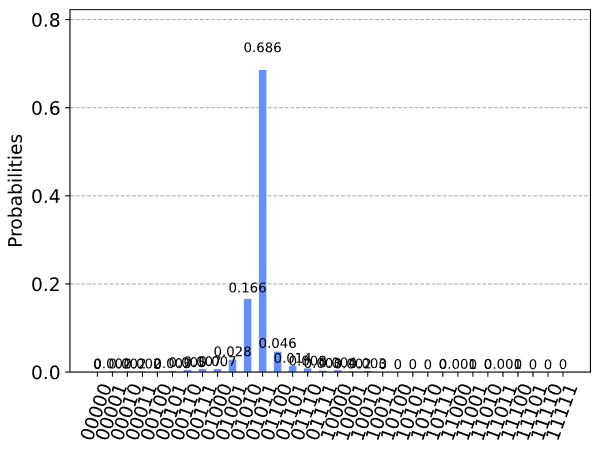\theta = \frac{11}{2^5} = 0.344,\;\text{ or }\;\; \theta = \frac{10}{2^5} = 0.313

## 4. 用真实设备进行实验

### 4.1 电路2.1


qpe.draw()





from qiskit.tools.monitor import job_monitor

provider = IBMQ.get_provider(hub='ibm-q')

santiago = provider.get_backend('ibmq_santiago')

# Run with 2048 shots

shots = 2048

t_qpe = transpile(qpe, santiago, optimization_level=3)

job = santiago.run(t_qpe, shots=shots)

job_monitor(job)




# get the results from the computation

results = job.result()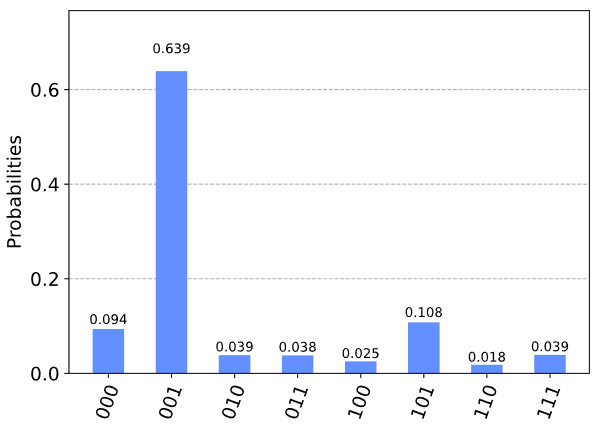## 5. 练习

1. 用不同的门尝试以上的实验( CNOT ，受控- S ，受控- T^\dagger )，你期望的结果是什么?你得到了什么结果?

2. 尝试受控- Y -门的实验，你得到了你想要的结果吗?(提示:请确保 |\psi\rangleY 的特征态!)

## 7. 参考文献

 Michael A. Nielsen and Isaac L. Chuang. 2011. Quantum Computation and Quantum Information: 10th Anniversary Edition (10th ed.). Cambridge University Press, New York, NY, USA.

## 8. 贡献者

03/20/2020 - HwajungKang (@HwajungKang) -修复了量子比特排序的不一致


import qiskit.tools.jupyter

%qiskit_version_table



### 版本信息

Qiskit Software Version
Qiskit 0.27.0
Terra 0.17.4
Aer 0.8.2
Ignis 0.6.0
Aqua 0.9.2
IBM Q Provider 0.14.0
System information
Python 3.7.7 (default, May 6 2020, 04:59:01) [Clang 4.0.1 (tags/RELEASE_401/final)]
OS Darwin
CPUs 8
Memory (Gb) 32.0

Wed Jun 16 09:47:53 2021 BST